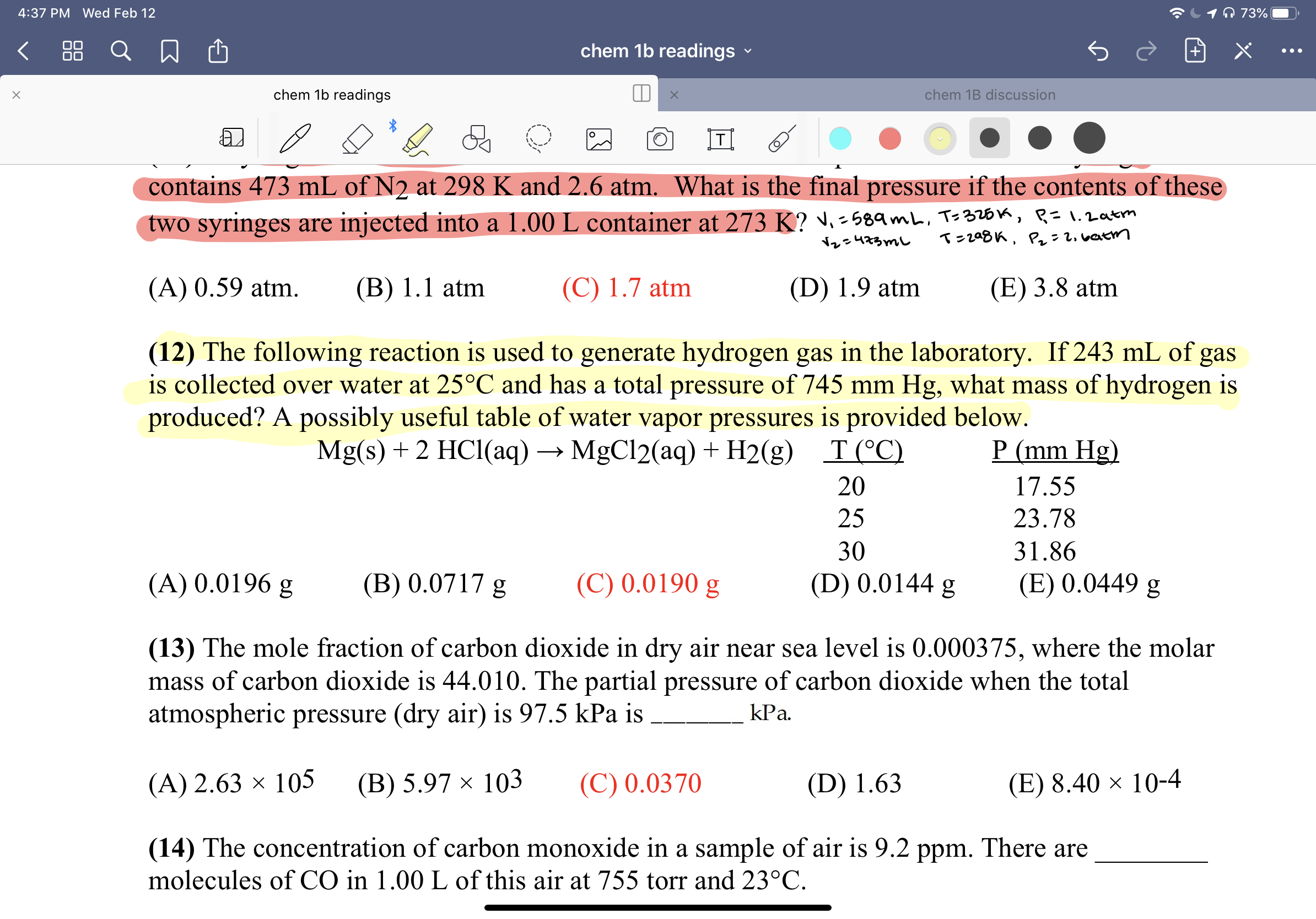# O 73%4:37 PM Wed Feb 1288chem 1b readingschem 1b readingschem 1B discussioncontains 473 mL of N2 at 298 K and 2.6 atm. What is the final pressure if the contents of thesetwo syringes are injected into a 1.00 L container at 273 K? ♥. -589mL, Ts326k, R=1.2atmT=208K, P: 2. vatmNレe473mし(A) 0.59 atm.(B) 1.1 atm(C) 1.7 atm(D) 1.9 atm(E) 3.8 atm(12) The following reaction is used to generate hydrogen gas in the laboratory. If 243 mL of gasis collected over water at 25°C and has a total pressure of 745 mm Hg, what mass of hydrogen isproduced? A possibly useful table of water vapor pressures is provided below.P (mm Hg)Mg(s) + 2 HCl(aq) → MgCl2(aq) + H2(g)T(°C)2017.552523.783031.86(C) 0.0190 g(A) 0.0196 g(B) 0.0717 g(D) 0.0144 g(E) 0.0449 g(13) The mole fraction of carbon dioxide in dry air near sea level is 0.000375, where the molarmass of carbon dioxide is 44.010. The partial pressure of carbon dioxide when the totalatmospheric pressure (dry air) is 97.5 kPa iskPa.(C) 0.0370(A) 2.63 × 105(В) 5.97 х 103(D) 1.63(E) 8.40 × 10-4(14) The concentration of carbon monoxide in a sample of air is 9.2 ppm. There aremolecules of CO in 1.00 L of this air at 755 torr and 23°C.

Question
1 views

I'm unsure of how to complete the question I attached. It's the question highlighted in yellow.help_outlineImage TranscriptioncloseO 73% 4:37 PM Wed Feb 12 88 chem 1b readings chem 1b readings chem 1B discussion contains 473 mL of N2 at 298 K and 2.6 atm. What is the final pressure if the contents of these two syringes are injected into a 1.00 L container at 273 K? ♥. -589mL, Ts326k, R=1.2atm T=208K, P: 2. vatm Nレe473mし (A) 0.59 atm. (B) 1.1 atm (C) 1.7 atm (D) 1.9 atm (E) 3.8 atm (12) The following reaction is used to generate hydrogen gas in the laboratory. If 243 mL of gas is collected over water at 25°C and has a total pressure of 745 mm Hg, what mass of hydrogen is produced? A possibly useful table of water vapor pressures is provided below. P (mm Hg) Mg(s) + 2 HCl(aq) → MgCl2(aq) + H2(g) T(°C) 20 17.55 25 23.78 30 31.86 (C) 0.0190 g (A) 0.0196 g (B) 0.0717 g (D) 0.0144 g (E) 0.0449 g (13) The mole fraction of carbon dioxide in dry air near sea level is 0.000375, where the molar mass of carbon dioxide is 44.010. The partial pressure of carbon dioxide when the total atmospheric pressure (dry air) is 97.5 kPa is kPa. (C) 0.0370 (A) 2.63 × 105 (В) 5.97 х 103 (D) 1.63 (E) 8.40 × 10-4 (14) The concentration of carbon monoxide in a sample of air is 9.2 ppm. There are molecules of CO in 1.00 L of this air at 755 torr and 23°C. fullscreen
check_circle

Step 1

The correct option is C).

Pressure exerted by Hydrogen gas at 25oC = Total Pressure – aqueous tension

where aqueous tension is the partial pressure of the water vapour present in the gas.

Pressure(P) of H2 gas &n...

### Want to see the full answer?

See Solution

#### Want to see this answer and more?

Solutions are written by subject experts who are available 24/7. Questions are typically answered within 1 hour.*

See Solution
*Response times may vary by subject and question.
Tagged in

### Chemistry